### Chatbot using nltk, NLP and tfidf vectorizer

Demand of virtual chat bots is increasing day by day, it serves many purposes but major one is reducing man-power. There are various approches to build a chat bot; 1. Intent and entitiy based (RASA). 2. Text and NLP based chat bot. 3.Dialogue based chatbot (GPT and GPT2).

We will demonstrate the text and NLP based approcah as it does not require much data and provides satisfing results in a very short period of time.

Python Code and explanation

Import the necessary libraries/modules.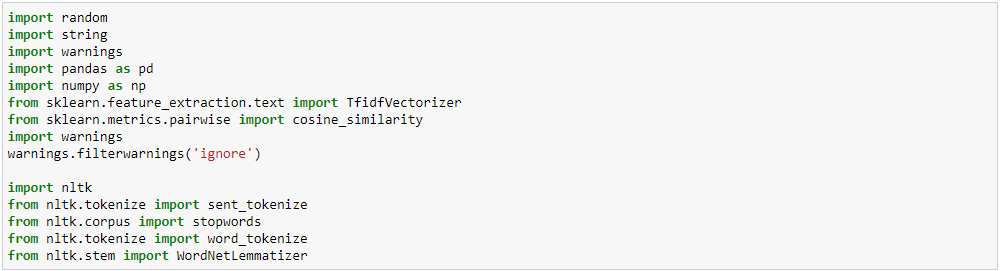Provide the raw data, represent it as question ::: answer format.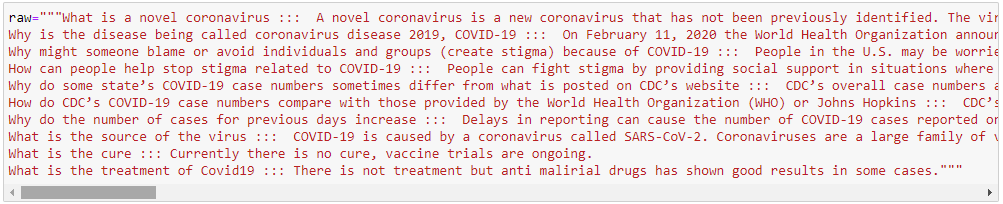Perform Contraction mapping : Contraction mapping means expanding the terms like I’ll to I will.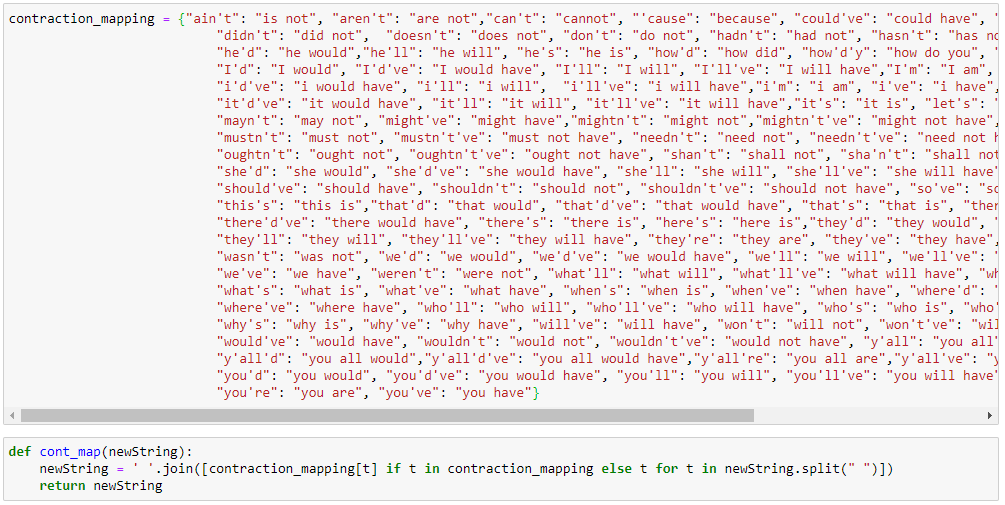Prepare separate lists of questions and answers, do the word tokenization of questions. Here we will tokenize and vectorize the questions only because a user will ask a question in this scenario. We will find out the cosine similarity between the user question and list of questions that we used to build the chat bot. We will return the best match if found else we will return a generic string.

Tokenization is the process of tokenizing or splitting a string, text into a list of tokens. One can think of token as parts like a word is a token in a sentence, and a sentence is a token in a paragraph.

`word_tokenize()` function is a wrapper function that calls tokenize() on an instance of the `TreebankWordTokenizer class`. These tokenizers work by separating the words using punctuation and spaces. And as mentioned in the code outputs above, it does not discard the punctuation, allowing a user to decide what to do with the punctuations at the time of pre-processing.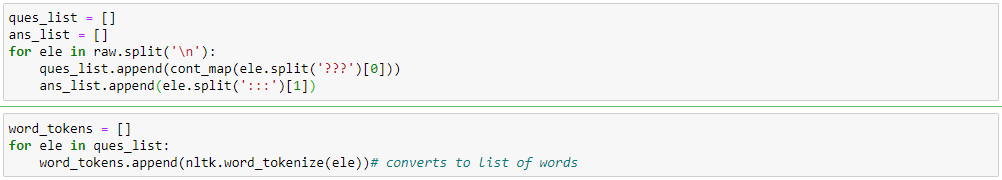Lemmatisation in linguistics is the process of grouping together the inflected forms of a word so they can be analysed as a single item, identified by the word’s lemma, or dictionary form. In layman term, we are finding the base word.
Examples:
rocks : rock
corpora : corpus
better : good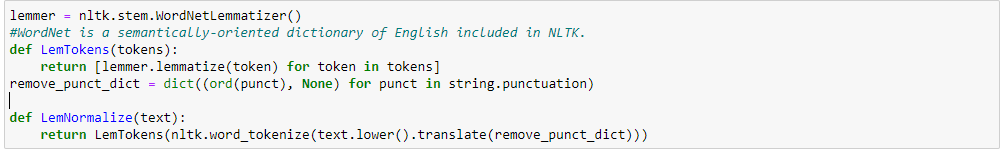Defining a function that replies on greeting.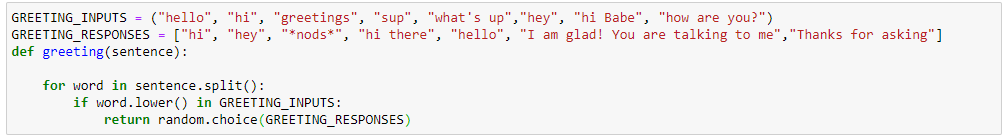Function to perform tfidf vectorization and cosine similarity. TFIDF was invented for document search and information retrieval. It works by increasing proportionally to the number of times a word appears in a document, but is offset by the number of documents that contain the word.

Cosine similarity is the cosine of the angle between two n-dimensional vectors in an n-dimensional space.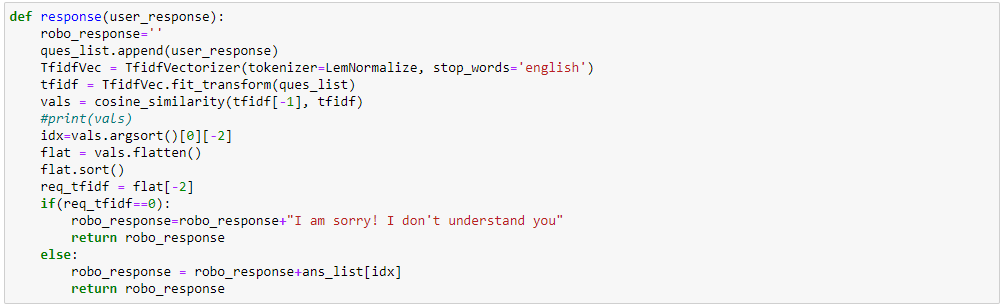Collaborating everything and calling the functions as per the user question/input.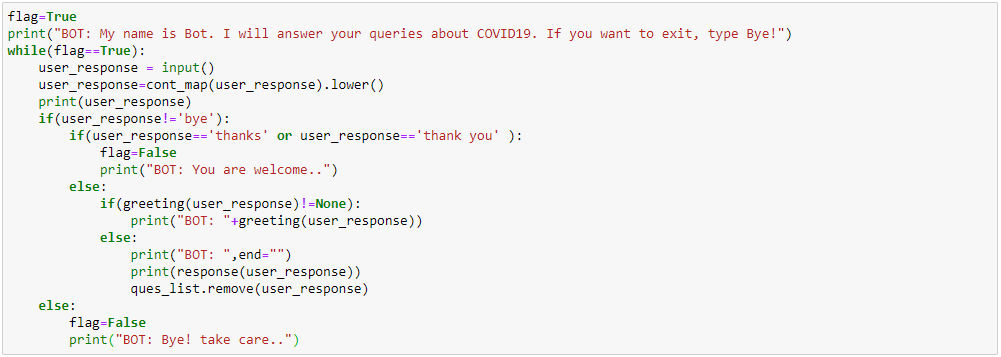Sample Output: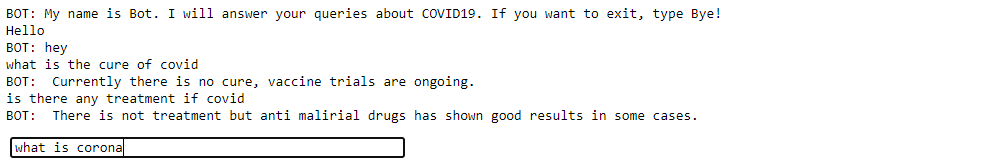Code:

### FLASK API to calculate WER, MER for text comparison in Python

Advance research is going on in the field of text analytics and NLP. Deep learning approach like Seq2Seq models and BERT have been established for tasks like language translation, abstractive text summarization and image captioning etc.
To determine how models are performing, we need performance metrics like rouge score, WER, blue score. WER or word error rate provides us a good vision by providing in depth analysis like words substituted, inserted or deleted.
Terminologies :

The original text is the reference text or the gold standard text, machine, or model generated text is the hypothesis test.

WER is formalized as (number of words present in machine-generated text but not in original text + number of words present in the original text but not in machine-generated text + number of words substituted) / (number of words in original text)

MER is formalized as (number of words present in machine-generated but not in original text + number of words present in the original text but not in machine-generated text) / (number of the word in machine-generated text)

HTML template :

How to run the code:

Folder Structure:
WER
— templates
— index.html
— wer.py

Make sure you have anaconda or python installed in your system with libraries flask, jiwer, numpy and re. Open anaconda prompt or terminal, navigate to WER folder and execute ‘python wer.py’. By default it will run on http://localhost:8018/ but you can change the port in wer.py file.

Test Result:

### Amazon Review Text Classification using Logistic Regression (Python sklearn)

Overview: Logistic Regression is the most commonly used classical machine learning algorithms. Although its name contains regression, it can be used only for classification. Logistic Regression can only be used for binary classification, but modified Logistic Regression can also be used for multiclass classification.

##### It has various advantages over other algorithms such as:
1. It has a really nice probabilistic interpretation, as well as geometric interpretation.
2. It is a parametric algorithm, and we need to store the weights that we learned during the training process to make predictions on the test data.
3. It is nothing but a linear regression function on which the Sigmoid Function has been applied to treat the outliers(or large values) in a better way.
1. Linear Regression Y = f(x)
2. Logistic Regression Y = sigmoid(f(x))
##### There are several assumptions while applying Logistic Regression on any dataset:
1. All the features are not multicollinear, and it can be tested using a perturbation test.
2. The dependent variable should be binary.
3. The dataset size should be large enough.
##### Logistic Regression Implementation on the Text Dataset (Using Sklearn):

You can download the data from here: https://www.kaggle.com/snap/amazon-fine-food-reviews First, we will clean the dataset. I have written a detailed post on the text data cleaning. You can read it here: https://kkaran0908.github.io/Text_Data_Preprocessing.html

After cleaning, we will divide the dataset into three parts, i.e., train, test, and validation set. Using the validation set, we will try to find out the optimal hyperparameters for the model. After getting optimal hyperparameter, we will test the model on the unseen data i.e. test set.

Now we vectorize the dataset using CountVectorizer (Bag of Words), it is one of the most straightforward methods to convert text data into numerical vector form.

Now we will import all the required that will be useful for the analysis.

Alpha, Penalty is the hyperparameters in Logistic Regression (there are others as well). We will try to find out the optimal values of these hyperparameters.

Output :

```0.0001 ------> 0.5
0.001  ------>  0.7293641972138972
0.01  ------>  0.8886922437232533
0.1  ------>  0.9374969316048458
1  ------>  0.9399004712804476
10  ------>  0.9113632222156819
100  ------>  0.8794308252229597
Optimal AUC score: 0.9399004712804476
Optimal C: 1```

“We can see that for c=1, we are getting an optimal AUC score, so for final modeling, we will use it.”

Our dataset we have two classes, so predict_proba(), is going to give us the probability of both the category. We can understand it by an example, so predict_proba() for a point will return the values like this [p,1-p], where p is the probability of positive point, and 1-p is the probability of the point being negative. For whichever category, we have a higher probability. We will assign that category to the test point.

OutPut:AUC score on test data: 0.8258208984684994

AUC score on the training data: 0.8909678471639081